# ISEE Middle Level Quantitative : Whole and Part

## Example Questions

### Example Question #1 : How To Find The Part From The Whole

Harvey bought a suit at a 25% employee discount at the store where he works. The suit originally cost $350.00. How much did he end up paying? Possible Answers: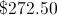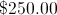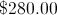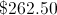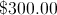Correct answer:Explanation: Finding 25% of a number is the same as multiplying it by 0.25; to get the discount, multiply 0.25 by the original purchase price of$350.00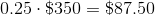To get the price Harvey paid, subtract the discount from the original price: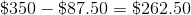### Example Question #211 : Numbers And Operations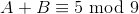Which of the following is true if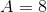?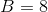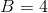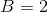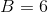Explanation:

Two expressions are equivalent in modulo 9 arithmetic if and only if, when each is divided by 9, the same remainder is yielded.,

so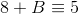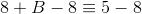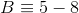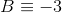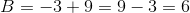, sois the correct choice.

### Example Question #3 : How To Find The Part From The Wholeis a positive integer. Which is the greater quantity?

(a) The remainder if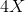is divided by 5

(b) The remainder if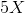is divided by 4

(b) is the greater quantity

(a) and (b) are equal

It is impossible to determine which is greater from the information given

(a) is the greater quantity

It is impossible to determine which is greater from the information given

Explanation:

The information is insufficient.

For example, if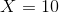: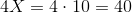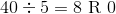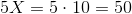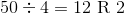This gives the division in (b) the greater remainder.

But if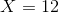: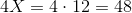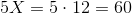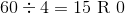This gives the division in (a) the greater remainder.

### Example Question #4 : How To Find The Part From The Wholeis a positive even integer. Which is the greater quantity?

(a) The remainder if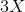is divided by 6

(b) The remainder if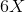is divided by 3.

(b) is the greater quantity

(a) is the greater quantity

(a) and (b) are equal

It is impossible to determine which is greater from the information given

(a) and (b) are equal

Explanation:

Sinceis an even integer, by definition, there is an integer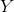such that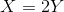.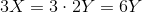; therefore,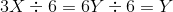; the remainder is 0.

Also,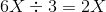; the remainder is 0.

The two remainders are both equal to 0.

### Example Question #5 : How To Find The Part From The Wholeis a positive odd integer. Which is the greater quantity?

(a) The remainder ifis divided by 8

(b) The remainder if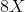is divided by 4

(b) is the greater quantity

(a) and (b) are equal

It is impossible to determine which is greater from the information given

(a) is the greater quantity

(a) is the greater quantity

Explanation: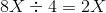, with a remainder of 0.

If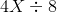were to yield a remainder of 0, then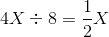must be a whole number; this can only happen ifis even. Sinceis odd, it follows that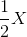is not a whole number, andmust yield a nonzero remainder. (a) must be the greater quantity.

### Example Question #1 : How To Find The Whole From The Part

150% of what number is 9,000?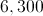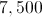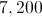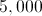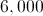Explanation:

Taking 150% of a number is the same as multiplying that number by 1.5. We can find our number, therefore, by dividing 9,000 by 1.5: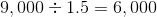### Example Question #2 : How To Find The Whole From The Partis a multiple of 2, but not a multiple of 6. Ifis divided by 6, What could be the remainder?Explanation:

If the remainder of dividingby 6 is 0, thenmust be a multiple of 6, which contradicts what we are given.

Suppose we call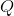andthe quotient and remainder, respectively, of dividingby 6. Then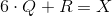If the remainder is 1, then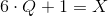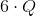, as a product of two integers, one even, is an even number; if 1 is added, the result is odd. Therefore, if 1 is the remainder,is odd, which also contradicts what we are given about. 1 cannot be the remainder, and for similar reasons, neither can 3.

This leaves 2, which can be the remainder; for example, 8, which is a multiple of 2, but not a multiple of 6, yields a quotient of 1 and a remainder of 2 when divided by 2.